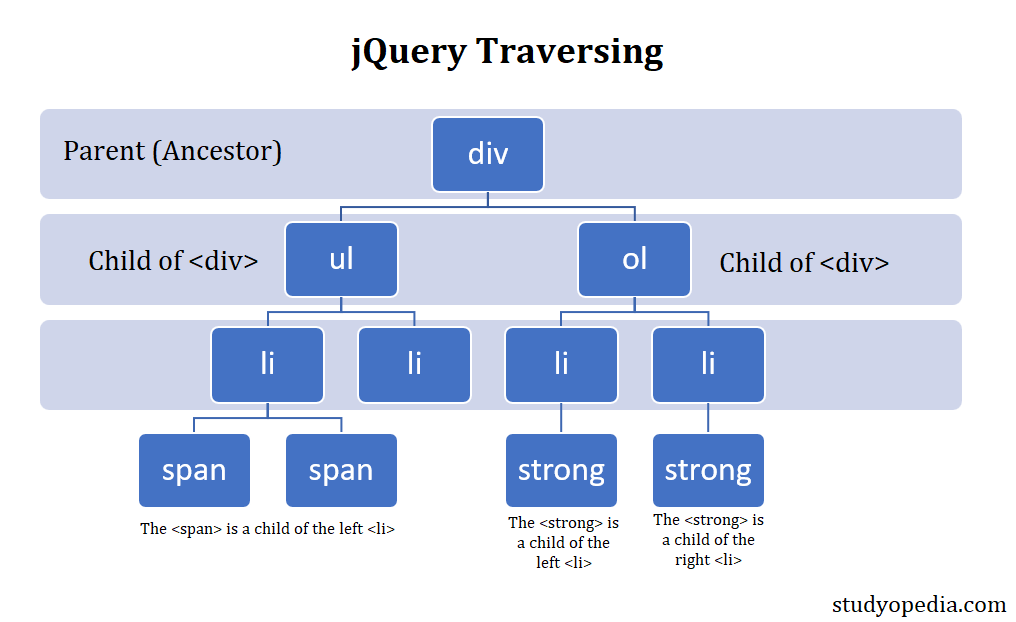## 27 Jan Introduction to jQuery Traversing

Consider an HTML web page as a DOM tree. Traversing means moving through the tree, like moving up, down, etc. Therefore, the need to understand the concept of Ancestors, Descendants, and Siblings is a must in jQuery. Consider the Ancestor is a parent, the Descendants are children, whereas the Siblings have similar parents.

Now, you must be wondering what DOM is. The Document Object Model (DOM) defines the logical structure of documents and the way a document is accessed and manipulated. It is a W3C (World Wide Web Consortium) standard.

Let us understand the traversing using the below figure:In the above figure:

• The <div> is the parent i.e., the ancestor.
• The <ul> is the left child of <div>.
• The <ol> is the right child of <div>.
• The <ul> and <ol> are siblings since they have the same parent.
• The <span> is a child of the left <li>
• The <strong> is a child of the left <li>.
• The <strong> is a child of the right <li>.

Let us now understand the Traversing concept in jQuery:[email protected]

We work to create programming tutorials for all.